# Lecture 15 - Directed graphs, DFS, DAGs, TopSort

Date Pre-lecture slides Post-lecture scribbles Lecture recording
October 17 2023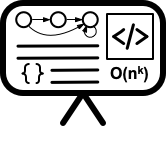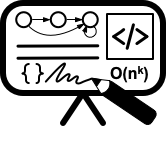## Notes

#### Directed Acyclic Graph

##### Definition

A directed graph G is a directed acyclic graph (DAG) if there is no directed cycle in G.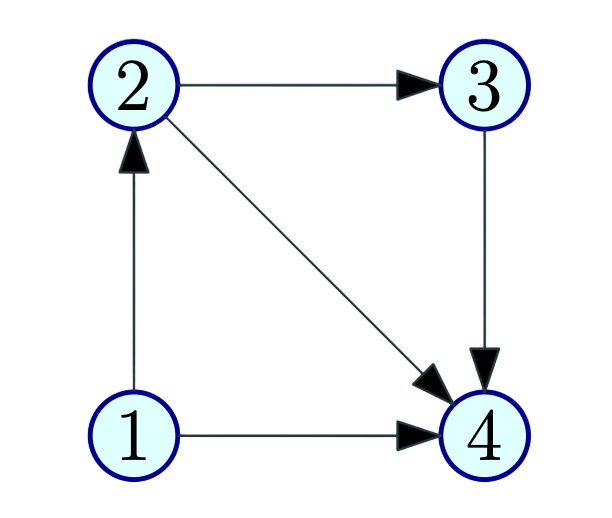• A vertex u is a source if it has no in-coming edges.
• A vertex u is a sink if it has no out-going edges.
##### Properties
• Every DAG G has at least one source and at least one sink
• G is a DAG if and only if $G^{rev}$ is a DAG
• G is a DAG if and only if each node is in its own strong connected component

#### Topological Ordering/Sorting

##### Definition

A topological ordering/topological sorting of G = (V, E) is an ordering $<$ on V such that if $(u → v) \in E$ then $u < v$ .

Informal Definition : One can order the vertices of the graph along a line (say the x-axis) such that all edges are from left to right.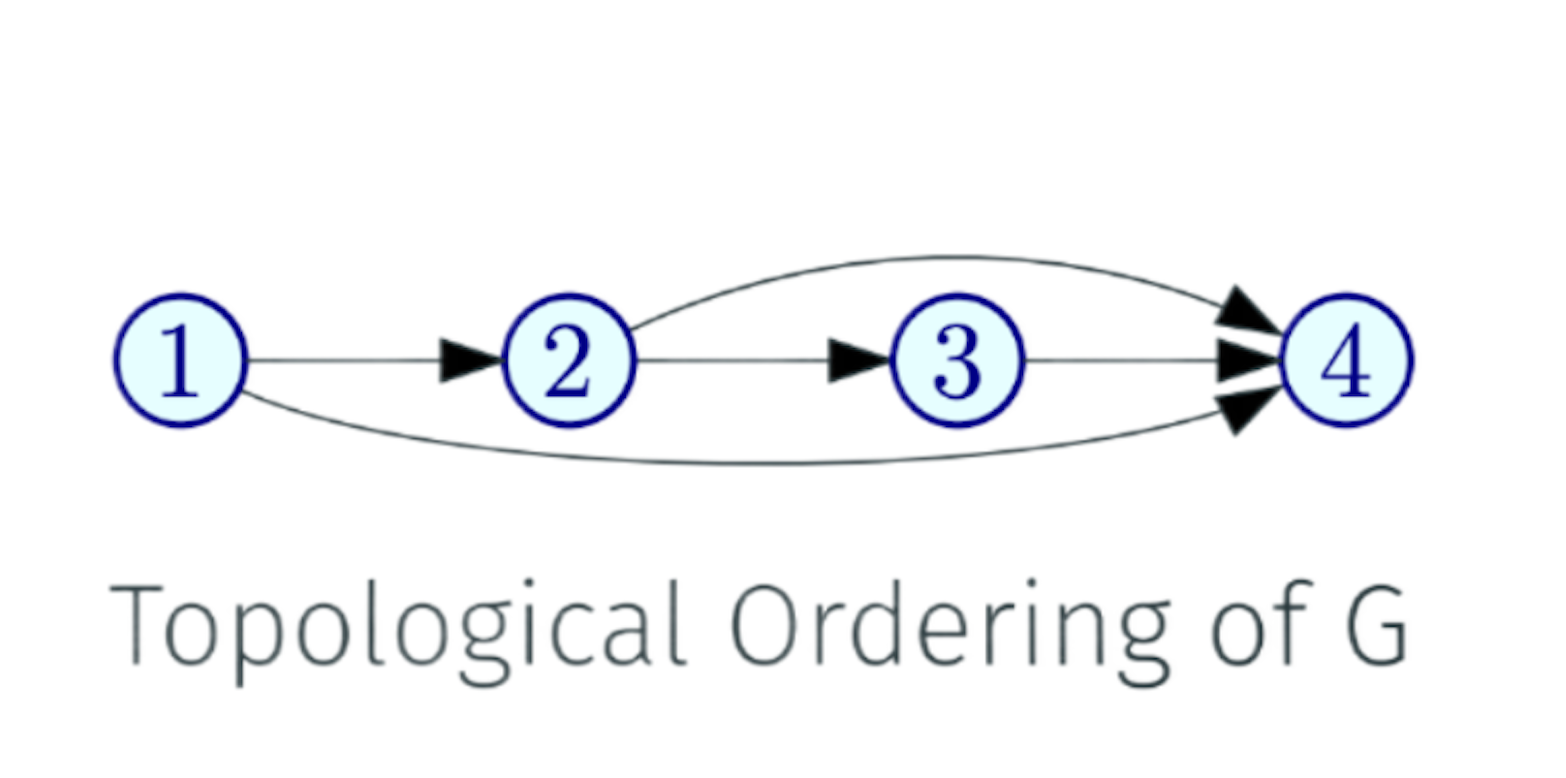• A DAG G may have many different topological sorts
• A directed graph G can be topologically ordered if G is a DAG
• A directed graph G is a DAG if G can be topologically ordered

#### Depth First Search

##### DFS with pre-post numbering in directed graphs

The Pre-visit number indicates when the node enters the DFS recursion stack, and the Post-visit number indicates when the node exits the DFS recursion stack. Pre and Post numbers can be used to determine whether a particular node is in the sub-tree of another node.

DFS(G)
Mark all nodes u as unvisited
T is set to ∅
time = 0
while there is an unvisited node u do
DFS(u)
Output T

DFS(u)
Mark u as visited
pre(u) = ++time
for each edge (u, v) in Out(u) do
if v is not visited
add edge (u, v) to T
DFS(v)
post(u) = ++time

##### Properties
• Node u is active in time interval [pre(u), post(u)]
• DFS(G) takes O(m + n) time
• Edges added form a branching: a forest of out-trees
• For any two nodes u and v, the two intervals [pre(u), post(u)] and [pre(v), post(v)] are disjoint or one is contained in the other
• To find whether u lies in the sub-tree of v or not we just compare the pre and post number of u and v. If $pre[u] > pre[v]$ and $post[u] < post[v]$ then u lies in the sub-tree of v otherwise not.
• Output of DFS(G) depends on the order in which vertices are considered
• If u is the first vertex considered by DFS(G) then DFS(u) outputs a directed out-tree T rooted at u and a vertex v is in T if and only if $v ∈ rch(u)$
##### Example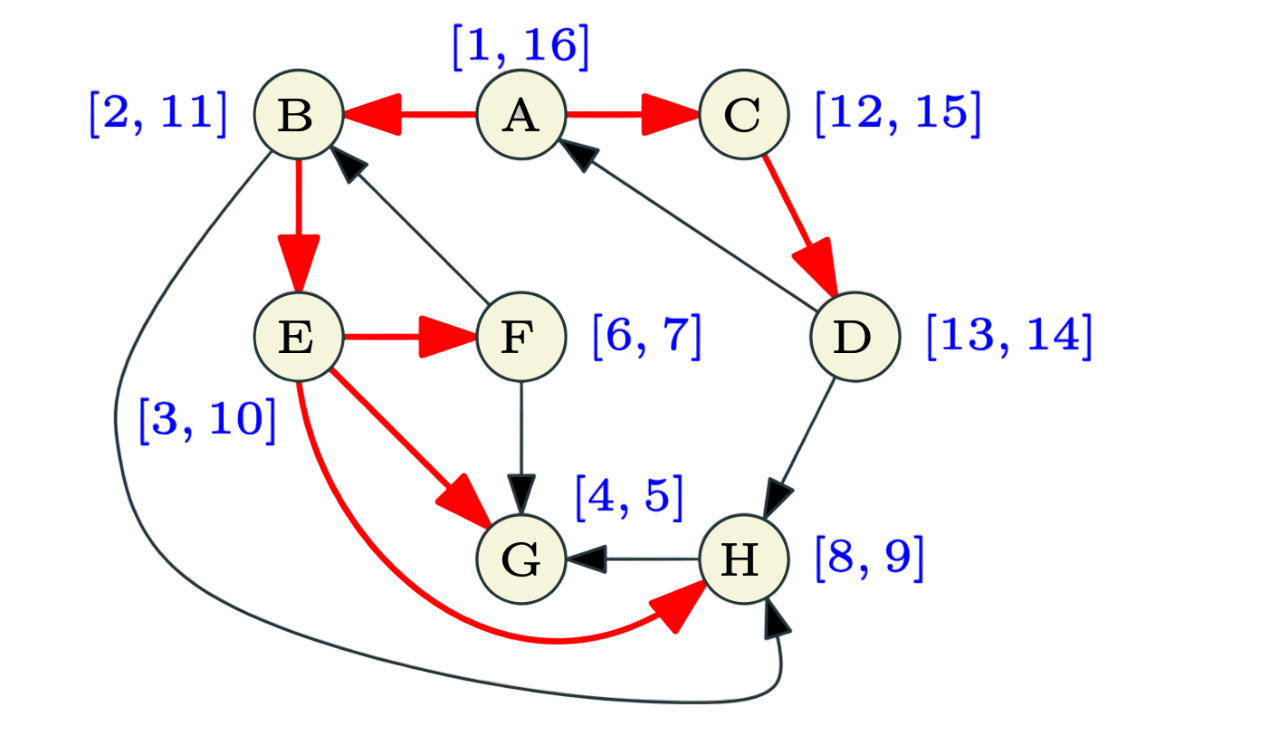Edges of G can be classified with respect to the DFS tree T as:

• Tree edges that belong to T
• A forward edge is a non-tree edges (x, y) such that y is a descendant of x i.e, $pre(x) < pre(y) < post(y) < post(x)$.
• A backward edge is a non-tree edge (x, y) such that y is an ancestor of x.
• A cross edge is a non-tree edges (x, y) such that they don’t have a ancestor/descendant relationship between them.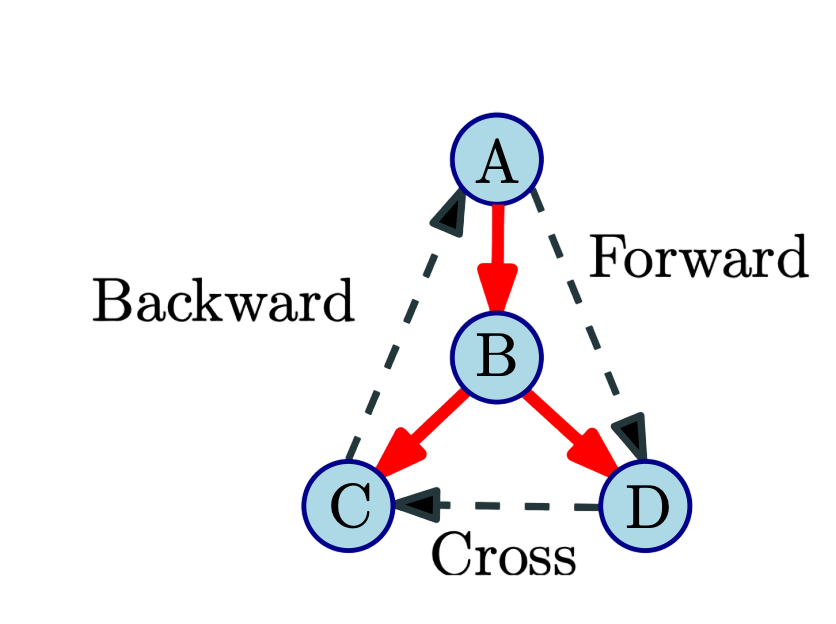Cycle detection in directed graph using topological sorting

Given a graph G, if it is a Directed Acyclic graph then compute a topological sort. If it failes, then output the cycle C.

The algorithm will be as follows:

• Compute DFS(G)
• If there is a back edge e = (v, u) then G is not a DAG. Output cycle C formed by path from u to v in T plus edge (v, u).
• Otherwise output nodes in decreasing post-visit order.

The above algorithm runs in $O(n + m)$ time.

#### Graph of strong connected components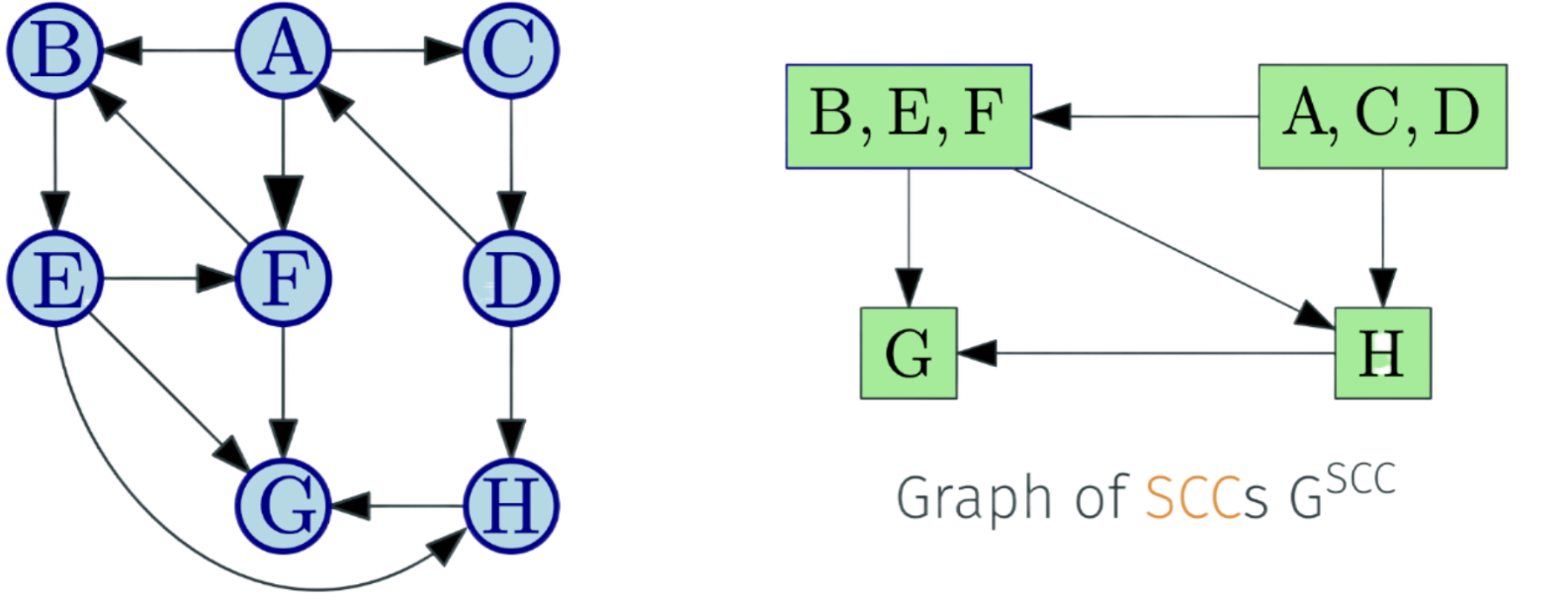Let $S_1, S_2, . . . S_k$ be the strong connected components (i.e.,SCCs) of G. The graph of SCCs is $G^{SCC}$. It is created by collapsing every strong connected component to a single vertex.

• Vertices are $S_1, S_2, . . . S_k$
• There is an edge $(S_i, S_j)$ if there is some $u \in S_i$ and $v \in S_j$ such that (u, v) is an edge in G

For a directed graph G, its meta-graph $G^{SCC}$ is a DAG.

The straightforward algorithm(discussed in Lecture 15) to find all SCCs of a given directed graph has a running time of $O(n(n + m))$.

The Linear time Algorithm for SCCs will be as follows:

• Let u be a vertex in a sink SCC of $G^{SCC}$
• Do DFS(u) to compute SCC(u)
• Remove SCC(u) and repeat

If v is the vertex with maximum post numbering in $DFS(G^{rev})$. Then v is in a SCC S, such that S is a sink of $G^{SCC}$. So, we can find a vertex in a sink SCC of $G^{SCC}$ for the linear time algorithm. Let us assume $G1=G^{rev}$.

do DFS(G1) and output vertices in decreasing post order.
Mark all nodes as unvisited
for each u in the computed order do
if u is not visited then
DFS(u)
Let S1 be the nodes reached by u
Output S1 as a strong connected component
Remove S1 from G


The above algorithm runs in time $O(m + n)$ and correctly outputs all the SCCs of G.

• Textbooks
• Erickson, Jeff. Algorithms
• Skiena, Steven. The Algorithms Design Manual
• Chapter 7.8 - Depth-First Search
• Chapter 7.9 - Applications of Depth-First Search
• Chapter 7.10 - Depth-First Search on Directed Graphs
• Sedgewick, Robert and Wayne, Kevin. Algorithms (Forth Edition)
• Chapter 4.2 - Directed Graphs
• Cormen, Thomas, et al. Algorithms (Forth Edition)
• Chapter 20.3 - Depth-First Search
• Chapter 20.4 - Topological Sort
• Sariel’s Lecture 16
• Jeff’s - Notes on Depth-First Search